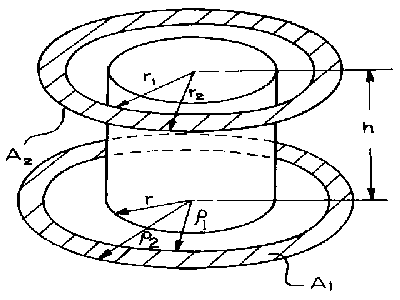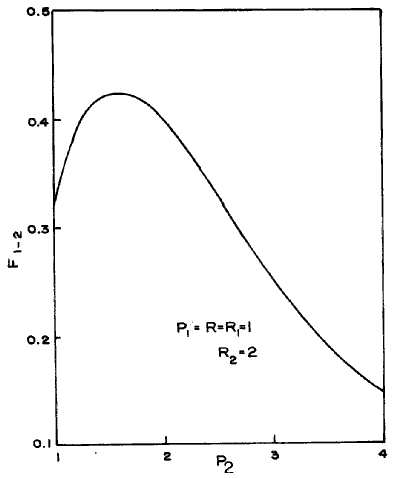# SECTION C Factors From Finite Areas to Finite Areas

## C-54: Coaxial annular rings separated by coaxial cylinder.

Reference: Minning (March, 1979)Definitions:

 P1 = r1/h;  P2 = r2/h; R1 = r1/h;  R2 = r2/h;   R = r/h

Graphical Results: